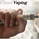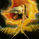14984 lượt xem
BB Support + Histo mode
-------------------------------
Code: http://pastebin.com/4QKdCEs7

Show enclosing BB

Show Insync as Histo:

v02 - Configurable levels
---------------------------------

Small update to allow configuring the 95/75/25/5 levels.

Latest source code: http://pastebin.com/fZ4PmemR

v01 - orginal description
---------------------------------

Insync Index, by Norm North, is a consensus indicator. It uses RSI , MACD , MFI , DPO , ROC , Stoch , CCI and %B to calculate a composite signal. Basically, this index shows that when a majority of underlying indicators is in sync, a turning point is near.

There are couple of ways to use this indicator.
- Buy when crossing up 5, sell when crossing down 95.
- Market is typically bullish when index is above 50, bearish when below 50. This can be a great confirmation signal for price action + trend lines .

Also, since this is typical oscillator, look for divergences between price and index.

Levels 75/25 are early warning levels. Note that, index > 75 (and less than 95) should be considered very bullish and index below 25 (but above 5) as very bearish . Levels 95/5 are equivalent to traditional OB/OS levels.

The various values of the underlying components can be tuned via options page. I have also provided an option to color bars based on the index value.

List of my free indicators: http://bit.ly/1LQaPK8
List of my app-store indicators: http://blog.tradingview.com/?p=970
(Support doc: http://bit.ly/lazybeardoc)
```//
// @author LazyBear
// List of all my indicators: http://bit.ly/1LQaPK8
//
study(title = "Insync Index [LazyBear]", shorttitle="II_LB")
src=close
div = input(10000, title="EMO Divisor", minval=1)
emoLength = input(14, minval=1, title="EMO length")
fastLength = input(12, minval=1, title="MACD Fast EMA Length")
slowLength=input(26,minval=1, title="MACD Slow EMA Length")
signalLength=input(9,minval=1, title="MACD Signal Length")
mfiLength = input(20, minval=1, title="MFI Length")

calc_emo() => sma(div * change(hl2) * (high - low) / volume, emoLength)
calc_macd(source) =>
fastMA = ema(source, fastLength)
slowMA = ema(source, slowLength)
fastMA - slowMA

calc_mfi(length) =>
src = hlc3
upper = sum(volume * (change(src) <= 0 ? 0 : src), length)
lower = sum(volume * (change(src) >= 0 ? 0 : src), length)
mf = rsi(upper, lower)
mf

calc_dpo(period_) =>
isCentered = false
barsback = period_/2 + 1
ma = sma(close, period_)
dpo = isCentered ? close[barsback] - ma : close - ma[barsback]
dpo

calc_roc(source, length) =>
roc = 100 * (source - source[length])/source[length]
roc

calc_stochD(length, smoothD, smoothK) =>
k = sma(stoch(close, high, low, length), smoothK)
d = sma(k, smoothD)
d

calc_stochK(length, smoothD, smoothK) =>
k = sma(stoch(close, high, low, length), smoothK)
//d = sma(k, smoothD)
k

lengthBB=input(20, title="BB Length"), multBB=input(2.0, title="BB Multiplier")
lengthCCI=input(14, title="CCI Length")
dpoLength=input(18, title="DPO Length")
lengthROC=input(10, title="ROC Length")
lengthRSI=input(14, title="RSI Length")
lengthStoch=input(14, title="Stoch Length"),lengthD=input(3, title="Stoch D Length"), lengthK=input(1, title="Stoch K Length")
lengthSMA=input(10, title="MA Length")

bolinslb=sma( src,lengthBB ) - multBB * ( stdev( src,lengthBB ) )
bolinsub=sma( src,lengthBB ) + multBB * ( stdev( src,lengthBB ) )
bolins2= (src- bolinslb ) / ( bolinsub - bolinslb )
bolinsll=( bolins2 < 0.05 ? -5 : ( bolins2 > 0.95 ? 5 : 0 ) )
cciins= ( cci(src, lengthCCI) > 100 ? 5 :  ( cci(src, lengthCCI ) < -100 ? -5 : 0 ) )
emvins2= calc_emo() - sma( calc_emo(),lengthSMA)
emvinsb= ( emvins2 < 0 ? ( sma( calc_emo() ,lengthSMA ) < 0 ? -5 : 0 ) : 0 )
emvinss= ( emvins2 > 0 ? ( sma( calc_emo() ,lengthSMA ) > 0 ? 5  : 0 ) : 0 )
macdins2= calc_macd( src) - sma( calc_macd( src) ,lengthSMA )
macdinsb= ( macdins2 < 0 ? ( sma( calc_macd( src),lengthSMA ) < 0 ? -5 : 0 ) : 0 )
macdinss=( macdins2 > 0 ? ( sma( calc_macd( src),lengthSMA) > 0 ? 5 : 0 ) : 0 )
mfiins=( calc_mfi( mfiLength ) > 80 ? 5 : ( calc_mfi( mfiLength ) < 20 ? -5 : 0 ) )
pdoins2=calc_dpo( dpoLength ) - sma( calc_dpo( dpoLength ),lengthSMA )
pdoinsb=( pdoins2 < 0 ? ( sma( calc_dpo( dpoLength ),lengthSMA) < 0 ? -5 : 0 ) :0 )
pdoinss=( pdoins2 > 0 ? ( sma( calc_dpo( dpoLength ),lengthSMA) > 0 ? 5 : 0 ) :0 )
rocins2=calc_roc( src,lengthROC  ) - sma( calc_roc( src,lengthROC ),lengthSMA  )
rocinsb=( rocins2 < 0 ? ( sma( calc_roc( src,lengthROC ),lengthSMA  ) < 0 ? -5 : 0 ) : 0 )
rocinss = ( rocins2 > 0 ? ( sma( calc_roc( src,lengthROC ),lengthSMA ) > 0 ? 5 : 0 ) : 0 )
rsiins= ( rsi(src, lengthRSI ) > 70 ? 5  : ( rsi(src, lengthRSI )  < 30 ? -5 : 0 ) )

stopdins=( calc_stochD(lengthStoch,lengthD,lengthK ) > 80 ? 5 : ( calc_stochD(lengthStoch,lengthD, lengthK ) < 20 ? -5 : 0 ) )
stopkins=( calc_stochK(lengthStoch,lengthD,lengthK) > 80 ? 5 : ( calc_stochK(lengthStoch,lengthD, lengthK ) < 20 ? -5 : 0 ) )

iidx = 50 + cciins + bolinsll + rsiins + stopkins + stopdins + mfiins + emvinsb + emvinss + rocinss + rocinsb + nz(pdoinss) + nz(pdoinsb ) + macdinss + macdinsb
ml=plot(50, color=gray, title="Line50")
ll=plot(5, color= green, title="Line5")
ul=plot(95, color = red, title="Line95")

plot(25, color= green, style=3, title="Line25")
plot(75, color = red, style=3, title="Line75")

fill(ml, ll, color=red)
fill(ml, ul, color=green)

il=plot(iidx, color=maroon, linewidth=2, title="InsyncIndex")
fill(ml,il,black)

bc = iidx >= 50 ? (iidx >= 95 ? #336600 : iidx >= 75 ? #33CC00 : #00FF00) :
(iidx <= 5 ? #990000 : iidx <= 25? #CC3300 :  #CC9900)

ebc = input(false, title="Enable Barcolors")
barcolor(ebc?bc:na)```
List of my free indicators: http://bit.ly/1LQaPK8
List of my indicators at Appstore: http://blog.tradingview.com/?p=970

## Bình luậnCame across this indicator by chance, been giving it a thorough test run, and damn!...this thing is deadly accurate (would seriously pay for this indicator to have it on my MT5). Thanks LazyBear...
Phản hồiThank you LazyBear ! Would it be possible to allow the thresholds to be user defined? 95/5 is a little restrictive for me; I might prefer 90/10 for OB/OS.
Tried to change the code, but didn't work for me......
Phản hồiTonyCampbell
Hi Tony

Here's the updated source code: http://pastebin.com/fZ4PmemR

This allows configuring Line95/75/25/5.

Thanks
Phản hồiLazyBear
Hello LazyBear

I get some kind of error message when I try to paste this into PineScript that says "mis-matched characters."